# Graphs of Cubic Functions

Mark Lewis, Benjamin Rosenthal
• Author
Mark Lewis

Mark has taught college and university mathematics for over 8 years. He has a PhD in mathematics from Queen's University and previously majored in math and physics at the University of Victoria. He has extensive experience as a private tutor.

• Instructor
Benjamin Rosenthal
What is a cubic function? See examples of cubic functions and learn how to graph cubic functions. Learn the equation and properties of a standard cubic function. Updated: 10/22/2021

Show

## What is a Cubic Function?

A cubic function is a polynomial of degree 3, meaning 3 is the highest power of {eq}x {/eq} which appears in the function's formula. The simplest example of such a function is the standard cubic function, which is simply

$$f(x) = x^3$$

Cubic functions often arise in situations where three factors are multiplied together, such as the volume of a rectangular box, which is equal to length times width times height. If each dimension is related to the variable {eq}x{/eq}, the result could be a cubic function something like this:

$$V(x) = l \times w \times h = x(6-2x)(10 -2x)$$

The function has degree 3 because if we expand it, by multiplying all terms involved, the highest power is the result of multiplying 3 {eq}x {/eq}'s. The graph of this function is shown below; as we will see, the graphs of most cubic functions have several basic features in common.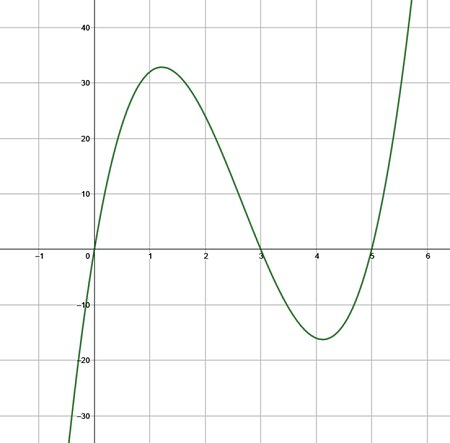### The Equation of a Cubic Function

The equations of cubic functions can always be expressed in the standard form

$$y= ax^3+bx^2+cx+d$$

where {eq}a, b, c, d {/eq} are real-valued constants. Remember that a cubic function is a polynomial whose highest degree term must be {eq}x^3{/eq}. This means that the coefficient {eq}a {/eq} must not be equal to 0. Lower powers may or may not be present, without changing the degree of the polynomial, so {eq}b, c, d {/eq} are allowed to be 0. Indeed, the standard cubic function does have {eq}b=c=d=0 {/eq} and {eq}a= 1 {/eq}.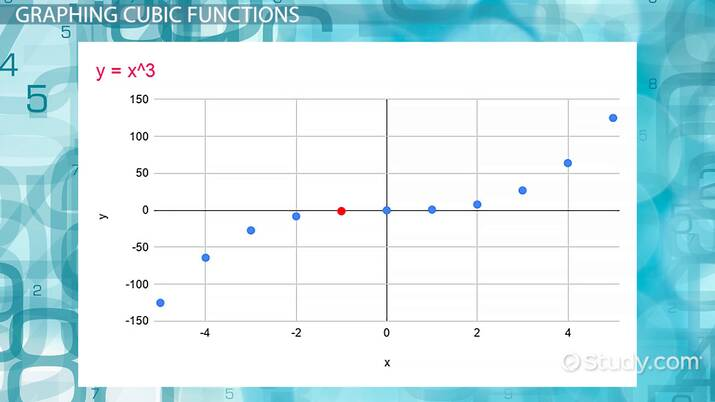An error occurred trying to load this video.

Try refreshing the page, or contact customer support.

Coming up next: Point Estimate in Statistics: Definition, Formula & Example

### You're on a roll. Keep up the good work!

Replay
Your next lesson will play in 10 seconds
• 0:04 Cubic Functions
• 0:49 Cubic Functions: Box Example
• 3:20 Cubic Functions: More Examples
• 4:40 Graphing Cubic Functions
• 6:50 Lesson Summary
Save Save

Want to watch this again later?

Timeline
Autoplay
Autoplay
Speed Speed

## How to Graph a Cubic Function

Cubic functions are more complicated than linear and quadratic functions, so it is often not easy to graph them based only on their equation in standard form. Creating a table of values and plotting points on the graph can be a good strategy, especially if we keep in mind one feature of cubic functions.

As an example, lets graph the cubic function

$$y=x^3 -8x^2 + 15 x + 1$$

To create a table of values, we can choose a range of {eq}x {/eq} values, and calculate corresponding values of {eq}y{/eq}. Choosing values close to 0, including a few negative values, is often the best place to start.

 x -2 -1 0 1 2 3 4 5 6 y -69 -23 1 9 7 1 -3 1 19

The points {eq}(x, y) {/eq} are plotted in the graph below.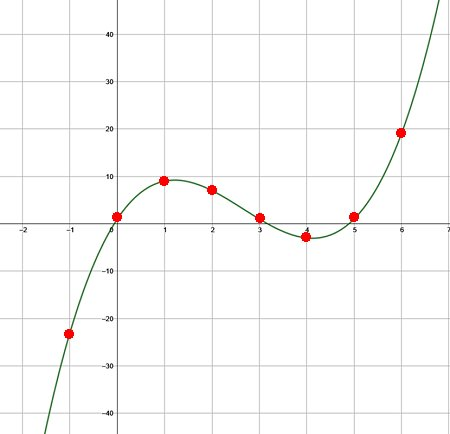Notice that the two graphs of cubic functions we have seen so far are very similar in shape. Both make a smooth, S-shaped curve that increases, then decreases, then increases again. Knowing this three-step pattern helps us accurately connect the dots to draw the graph of a cubic function. Also, seeing this pattern in the {eq}y {/eq} values in the table tells us that we have identified enough points to begin drawing the graph.

### X-Intercepts and Y-Intercepts

The points where a graph crosses the horizontal and vertical axes are called {eq}x {/eq}-intercepts and {eq}y {/eq}-intercepts. These make great reference points when graphing a function, and conversely, recognizing them in a graph can help us to identify the function's equation.

Can you identify the intercepts of the cubic function graphed below?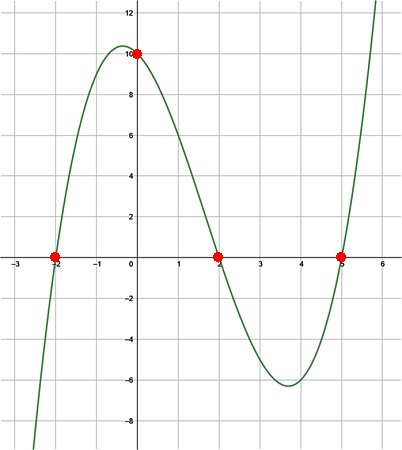If a cubic function has three {eq}x {/eq}-intercepts, or roots, {eq}r_1 {/eq}, {eq}r_2 {/eq}, and {eq}r_3 {/eq}, we can express its equation in the factored form

$$y = a (x-r_1)(x-r_2)(x-r_3)$$

The other constant {eq}a {/eq} can then be determined from the value of the {eq}y{/eq}-intercept.

The function in the graph above appears to cross the vertical axis at {eq}y=10 {/eq} and the horizontal axis at {eq}x=-2, 2, 5 {/eq}. Using these three roots, we can write the function as

$$y = a (x+2)(x-2)(x-5)$$

The graph reaches the {eq}y {/eq}-intercept of 10 when {eq}x=0 {/eq}, so it must be that

$$\begin{eqnarray} 10 &=& a (0+2)(0-2)(0-5) \\ 10& = & a (2)(-2)(-5) \\ 10 &=& 20 a \\ a &=& 0.5 \end{eqnarray}$$

The complete equation of the cubic function in factored form is

$$y = 0.5 (x+2)(x-2)(x-5)$$

It's important to note that not all cubic functions have three roots. Some may have two (including a double root), or even only one. For cubic functions with a single root, we won't be able to express the equation as a product with three linear factors.

### Constants

The exact shape of a cubic function is completely determined from the values of the constants {eq}a, b, c, d {/eq} in its standard form equation. Two of these constants tell us particularly useful information about the shape of the graph.

The constant {eq}d {/eq} determines the {eq}y {/eq}-intercept, the value of the function when {eq}x=0 {/eq}.

The other important constant is the leading coefficient {eq}a {/eq}, which appears in both the standard and factored equations. This coefficient is a "stretch factor" which can make the graph taller and narrower if {eq}|a|>1 {/eq}, or wider and shallower if {eq}|a|<1 {/eq}.

If {eq}a {/eq} is a negative value, then the graph is flipped, or reflected across the {eq}x {/eq}-axis. In this case, the three-step pattern in the graph is reversed: the graph will decrease, then increase, then decrease again. Its important to identify the sign of {eq}a {/eq} and the corresponding three-step pattern when graphing a cubic function, or identifying its equation.

There is one group of cubic functions that actually don't show the three-step pattern at all, and the standard cubic, shown below, is one of them!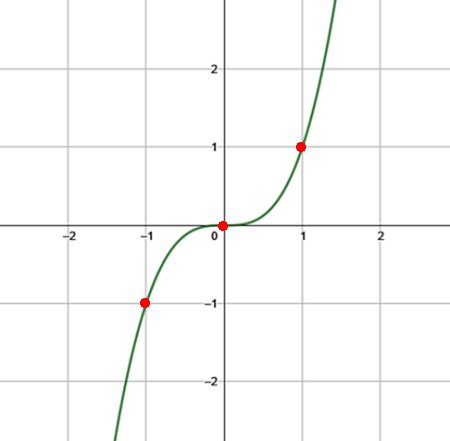The standard cubic function passes through the origin {eq}(0,0) {/eq} and is always increasing, without forming the distinctive peak-and-valley shape of the previous graphs. Two adjacent points on the graph are easily identified at {eq}(1, 1) {/eq} and {eq}(-1, -1) {/eq}.

Some cubic equations consist of transformations of the standard function and will have the same basic shape. Their equations can be expressed in the form

$$y=a(x-h)^3+k$$

The constant {eq}a {/eq} is the same stretch/reflection as before, while {eq}h {/eq} and {eq}k {/eq} define horizontal and vertical translations of the parent function {eq}y= x^3 {/eq}.

To unlock this lesson you must be a Study.com Member.

#### How do you find the equation of a cubic graph?

If a cubic graph has three x-intercepts then it is possible to quickly express the equation in factored form. The leading coefficient can be determined from the y-intercept.

#### How do you translate a cubic function?

A translation of the standard cubic function, y=x^3, takes the form y=a(x-h)^3+k. The constant h is a horizontal translation to the right, and k is a vertical translation upwards.

#### What is the equation for a cubic function?

The equation of a cubic function can always be expressed in the standard form y=ax^3+bx^2+cx+d, where a, b, c, d are constants, with a non-zero.

#### How do you graph a cubic function?

In many cases, a cubic function is most easily graphed by creating a table of values and plotting the points. If the equation can be factored, it is instead possible to graph it by locating its x- and y-intercepts.

### Register to view this lesson

Are you a student or a teacher?